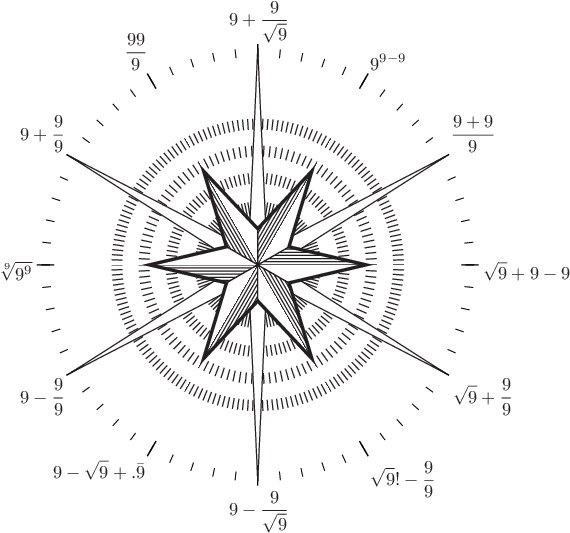# Pyxplot

## Examples - A clockDownload this example:

Example plot - a novelty clock

In this example script, we use Pyxplot's text command and stars plotting style to produce a novelty clock where all of the numerals are made up from three nines.

### Script

# Make an eps image of a star to go in the centre of the image

set term eps
set out 'star.eps'
set axis x invisible
set axis y invisible
set nokey
plot '--' with stars
0 0
END
clear

# Now make the clock proper

set output 'output.eps'

set fontsize 1.2

w          = 10
w2         = w/2
eps_margin = 0.2

rot(theta) = vector(sin(theta),cos(theta))

set multiplot ; set nodisplay

# Make the numbers from 1 to 12

set texthalign centre
text r"\parbox{2in}{$9+\frac{9}{\sqrt{9}}$}" at p(0)

set texthalign left
text r"\parbox{2in}{$9^{9-9}$}" at p(pi/6)
text r"\parbox{2in}{$\frac{9+9}{9}$}" at p(pi/3)

set textvalign centre
text r"\parbox{2in}{$\sqrt{9}+9-9$}" at p(pi/2)

set textvalign top
text r"\parbox{2in}{$\sqrt{9}+\frac{9}{9}$}" at p(2*pi/3)
text r"\parbox{2in}{$\sqrt{9}!-\frac{9}{9}$}" at p(5*pi/6)

set texthalign centre
text r"\parbox{2in}{$9-\frac{9}{\sqrt{9}}$}" at p(pi)

set texthalign right
text r"\parbox{2in}{$9-\sqrt{9}+.\bar{9}$}" at p(7*pi/6)
text r"\parbox{2in}{$9-\frac{9}{9}$}" at p(4*pi/3)

set textvalign centre
text r"\parbox{2in}{$\sqrt{9^{9}}$}" at p(3*pi/2)

set textvalign bottom
text r"\parbox{2in}{$9+\frac{9}{9}$}" at p(10*pi/6)
text r"\parbox{2in}{$\frac{99}{9}$}" at p(11*pi/6)

for i = 0 to 2*pi step pi/30
{
arrow from w/2*rot(i) to (w2-eps_margin)*rot(i) w nohead
}

for i = 0 to 2*pi step pi/6
{
arrow from (w2+    eps_margin/2)*rot(i) \
to   (w2-1.5*eps_margin  )*rot(i) w nohead lw 1.5
}

set texthalign centre
set textvalign centre
eps 'star.eps' at 0,0 wid w/2 rot -10

set display ; refresh